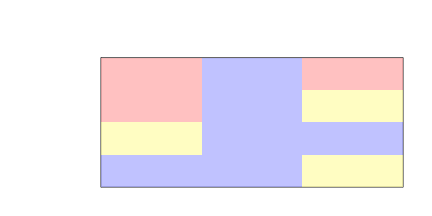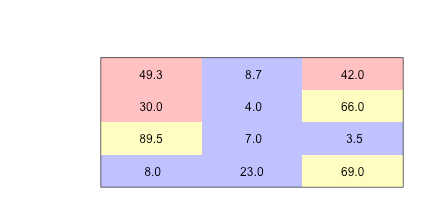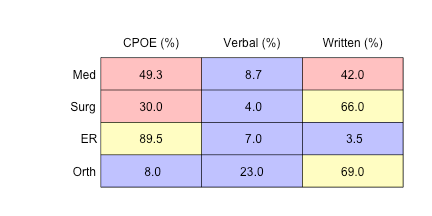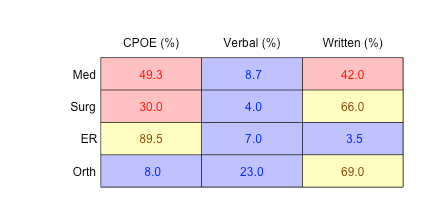Want to share your content on R-bloggers? click here if you have a blog, or here if you don't.

## Background

There are a few ways to approach the problem of a conditionally formatted table in R. You can use the ReporteRs package's `FlexTable()` function, the formattable package, or the condformat package. These allow you to produce a conditionally formatted tables in HTML. You can also use xtable package and essentially program what you want in LaTeX via the `xtable()` function.

In my desire for something simple-ish, I am going do this graphically using the `image()` function as suggested here. The benefit is that I can then push the table into an RMarkdown generated PDF document easily.

## The Problem

Suppose that you want to prepare a summary of how resident and medical student orders are placed on various wards. You obtain data that is formatted in the following manner.

`head(orders,10)`

```##    ward order.type cosigned
## 1   Med       CPOE     TRUE
## 2   Med    Written    FALSE
## 3   Med       CPOE     TRUE
## 4   Med    Written     TRUE
## 5   Med    Written     TRUE
## 6   Med    Written     TRUE
## 7   Med       CPOE     TRUE
## 8   Med       CPOE    FALSE
## 9   Med       CPOE     TRUE
## 10  Med       CPOE     TRUE```

There are 4 wards: medicine, surgery, ER and orthopedics. Orders can come in as computerized physician order entry (CPOE), verbal or written. The orders have to be cosigned by staff and this is recorded as TRUE/FALSE because staff are not always compliant in logging on to the EMR to cosign the trainee orders.

`str(orders)`

```## 'data.frame':    550 obs. of  3 variables:
##  \$ ward      : Factor w/ 4 levels "Med","Surg","ER",..: 1 1 1 1 1 1 1 1 1 1 ...
##  \$ order.type: Factor w/ 3 levels "CPOE","Verbal",..: 1 3 1 3 3 3 1 1 1 1 ...
##  \$ cosigned  : logi  TRUE FALSE TRUE TRUE TRUE TRUE ...```

`summary(orders)`

```##    ward       order.type   cosigned
##  Med :150   CPOE   :291   Mode :logical
##  Surg:100   Verbal : 54   FALSE:195
##  ER  :200   Written:205   TRUE :355
##  Orth:100                 NA's :0```

## Preparing Proportions Table

Let's start with the assumption that we want to apply the same conditional formatting to all data in the table. That is, we want to color code all results with the same algorithm. We can used the `image()` function to get this done. Let's display the rates at which different order types (CPOE, verbal,or written) from the four wards. We can generate the proportions table in percent very easily with the `prop.table()` and `table()` functions operating on the first two columns of our `orders` data:

```my.data <- round(prop.table(table(orders[,1:2]),1)*100,1)
my.data```

```##       order.type
## ward   CPOE Verbal Written
##   Med  49.3    8.7    42.0
##   Surg 30.0    4.0    66.0
##   ER   89.5    7.0     3.5
##   Orth  8.0   23.0    69.0```

## A DIY Approach with the Image Function

The `image()` function produces a tile plot based on matrix of z values, where z = f(x,y) using colours we can define and thresholds for switching from one colour to the next based on a `breaks` parameter. In our case, we will say that if the result is less than equal to 25%, we will colour the tile blue, if it is greater than 25% but less than or equal to 50%, we will colour it red, and if it greater than 50%, it will be yellow.

You will note that we have to transpose the data with the `t()` function because the image function plots the rows on the x axis on the columns on the y axis. You will also notice that we need to plot y descending on the y-axis to account for the fact that our tabular data has increasing index going down but the tile plot will default to have increasing y going up. We can also need to suppress the axes and their labels. The reader can comment out the lines `xaxt = 'n'` and `yaxt = 'n'` to see what is going on in terms of x and y values.

```x = 1:ncol(my.data)
y = 1:nrow(my.data)
centers <- expand.grid(y,x)

#make the plot margins a little bigger
par(mar = c(2,7,4,2))

image(x, y, t(my.data),
col = c(rgb(0,0,1,0.3),rgb(1,0,0,0.3), rgb(1,1,0,0.3)),
breaks = c(0, 25, 50, 100),
xaxt = 'n',
yaxt = 'n',
xlab = '',
ylab = '',
ylim = c(max(y) + 0.5, min(y) - 0.5)
)```Now we can write our values over top with the `text()` function.

`text(centers[,2], centers[,1], c(my.data), col= "black")`And then we can write the variable names (which we yank from the attributes of the table) into the figure margin and draw some lines to make it look pretty. It was necessary to use the `adj` and `padj` parameters to make it look a little cleaner.

```#add margin text
mtext(attributes(my.data)\$dimnames[], at=1:nrow(my.data), side = 2, las = 1, adj = 1.2)

abline(h=y + 0.5)
abline(v=x + 0.5)```## Conditionally Coloured Text

Now, if you want to make the text colour match the background colour, we will need a little function.

```color.picker <- function(z){
if(z <= 25){return("blue")}
else if( z > 25 & z <= 50){return("red")}
else {return("darkorange4")}
}```

and then apply it over the values of the matrix:

```text.cols <- sapply(c(my.data), color.picker)
text(centers[,2], centers[,1], c(my.data), col= text.cols)```## Different Conditions for Different Columns

Now suppose you wanted different conditional formatting for each column. This is kind of a pain because you will need to provide the `image()` function a matrix to generate an appropriate fill-colour and a different matrix for the data to be written in each cell. Let's imagine for example that we want to include the compliance rate for co-signing in a fourth column and this is the only column we want coloured. To this column we want a colour scheme applied wherein if compliance is less than or equal to 20%, the colour is red, between 20% and 80%, it is yellow, and above 80% it is green.

We can calculate a proportions table based on columns 1 and 3 of the `orders` dataframe and then we can define a matrix `fill.data` that has `NA` on all the rates we calculated above.

```my.data <- cbind(my.data,Cosigned = round(prop.table(table(orders[,c(1,3)]),1)*100,1)[,2])
fill.data <- my.data
fill.data[,1:3] <- matrix(NA, nrow = nrow(my.data), ncol = ncol(my.data) - 1)```

Now the proportions matrix is as follows:

`my.data`

```##      CPOE Verbal Written Cosigned
## Med  49.3    8.7    42.0     75.3
## Surg 30.0    4.0    66.0     52.0
## ER   89.5    7.0     3.5     88.5
## Orth  8.0   23.0    69.0     13.0```

and the fill data is:

`fill.data`

```##      CPOE Verbal Written Cosigned
## Med    NA     NA      NA     75.3
## Surg   NA     NA      NA     52.0
## ER     NA     NA      NA     88.5
## Orth   NA     NA      NA     13.0```

Now we can apply the `image()` function to the `fill.data` matrix. When it comes to writing the data in the cells, we will use the original `my.data` matrix and we will adjust out `color.picker()` function.

```color.picker <- function(z){
if(is.na(z)){return("black")}
else if(z <= 20){return("red")}
else if( z > 20 & z <= 80){return("darkorange4")}
else {return("darkgreen")}
}

x = 1:ncol(my.data)
y = 1:nrow(my.data)
centers <- expand.grid(y,x)

par(mar = c(2,7,4,2))
image(x, y, t(fill.data),
col = c(rgb(1,0,0,0.3),rgb(1,1,0,0.3), rgb(0,1,0,0.3)),
breaks = c(0, 20, 80, 100),
xaxt='n',
yaxt='n',
xlab='',
ylab='',
ylim = c(max(y) + 0.5, min(y) - 0.5)
)

#write in values
text.cols <- sapply(c(fill.data), color.picker)
text(centers[,2], centers[,1], format(c(my.data),nsmall = 1), col= text.cols)

mtext(attributes(my.data)\$dimnames[], at=1:nrow(my.data), side = 2, las = 1, adj = 1.2)

abline(h=y + 0.5)
abline(v=x + 0.5)```So, it looks like this could become super–awkward if we had elaborate conditions to apply. This is where a packages like condformat and formattable come in handy. If you use the condformat package, you can include the table in an RMarkdown generated PDF or HTML document. However, the `formattable()` function, though capable of much prettier output, does not work with PDFs generated using RMarkdown.

First, here is a condformat example. Suppose we wanted to colourized CPOE in shades of green because CPOE is more operationally desirable and verbal/written orders in shades of red because they are less operationally desirable. We also want the red/yellow/green formatting in the Cosigned column. Using condformat we could do the following:

```library(condformat)

my.data <- as.data.frame(my.data)

color.picker <- function(z){
if(is.na(z)){return(0)}
else if(z <= 20){return(1)}
else if( z > 20 & z <= 80){return(2)}
else {return(3)}
}

condformat(my.data) +
rule_fill_gradient(CPOE, low = rgb(1,1,1), high = rgb(0,1,0)) +
rule_fill_gradient(Verbal, low = rgb(1,1,1), high = rgb(1,0,0)) +
rule_fill_gradient(Written, low = rgb(1,1,1), high = rgb(1,0,0)) +
rule_fill_discrete(Cosigned, expression = sapply(Cosigned,
color.picker),colours=c("0" = "white", "1" = "red",
"2" =  "yellow", "3" = "lightgreen"))```

CPOEVerbalWrittenCosigned
149.3 8.742.075.3
230.0 4.066.052.0
389.5 7.0 3.588.5
4 8.023.069.013.0

You can see that the rownames are suppressed with `condformat()`. You could circumvent this by putting the rownames into their own column. This package is pretty easy to use and with PDF rendering (shown below) it produces something more LaTeX-ish than what is shown above which was generated straight to HTML.For something more attractive looking, here is an example of something similar using the formattable package (borrowing heavily from the code author's examples ):

```library(formattable)

color.picker <- function(z){
if(is.na(z)){return("black")}
else if(z <= 20){return("red")}
else if( z > 20 & z <= 80){return("darkorange")}
else {return("darkgreen")}
}

bg.picker <- function(z){
if(is.na(z)){return("black")}
else if(z <= 20){return("pink")}
else if( z > 20 & z <= 80){return("yellow")}
else {return("lightgreen")}
}

my.data <- as.data.frame(my.data)

formattable(my.data, list(
CPOE = color_tile("white", "green"),
Verbal = color_tile("white", "red"),
Written = color_tile("white", "red"),
Cosigned = formatter("span",
style = x ~ style(display = "block",
color = sapply(x,color.picker),
"background-color" = sapply(x,bg.picker)),
x ~ sprintf("%.2f (rank: %02d)", x, rank(-x)))
))```

CPOE Verbal Written Cosigned
Med 49.3 8.7 42.0 75.30 (rank: 02)
Surg 30.0 4.0 66.0 52.00 (rank: 03)
ER 89.5 7.0 3.5 88.50 (rank: 01)
Orth 8.0 23.0 69.0 13.00 (rank: 04)

I hope that this points you in the right direction.

And as for conditions:

“If you declare with your mouth, “Jesus is Lord,” and believe in your heart that God raised him from the dead, you will be saved.”

Romans 10:9